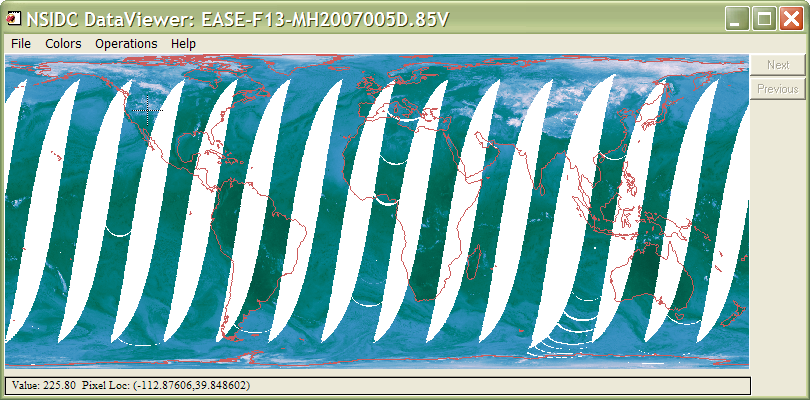Faking a Cylindrical Equal-Area Map Projection

QUESTION: Many images downloaded from the National Snow and Ice Data Center (NSIDC) archives are projected onto a Behrmann Cylindrical Equal-Area map projection. I would like to display land boudaries and map grids on such an image, but I can't seem to find a Cylindrical Equal-Area map projection available in IDL. What can I do?ANSWER: The best thing you can do is write a letter to IDL Technical Support asking them to include this map projection in future versions of IDL. The second best thing you can do is cobble together a fake cylindrical projection. This article explains how I have done this in the past to obtain reasonable results.

One such NSIDC data set is the SSM/I Microwave Global Brightness Temperatures. This is gridded into a Behrmann Cylindrical Equal-Area map projection with a longitude range of -180.0 to 180, a latitude range of -86.72 to 86.72, and a true scale at plus and minus 30 degrees of latitude. The radius of the Earth sphere is given as 6371228 meters. Since IDL does not give the user access to a cylindrical equal-area projection, I have tried to solve this problem by using an Albers Equal-Area Conic projection from the CGTP map projection toolbox, accessed from within IDL using Map_Proj_Init. If I set the true-scale parallels in this projection to about plus or minus 60 degrees, I can achieve a reasonable approximation to a cylindrical projection.

I set the projection up like this.

mapStruct = Map_Proj_Init(103, \$  ; Albers Equal-Area Conic Projection
SEMIMAJOR_AXIS=6371228L, \$         SEMIMINOR_AXIS=6371228L,\$
CENTER_LONGITUDE=0.0, \$         CENTER_LATITUDE=0.0, \$
STANDARD_PAR1=60, \$         STANDARD_PAR2=-59.5, \$
LIMIT=[-86.72, -180, 86.72,  180])    xrange = mapStruct.uv_box[[0,2]]
yrange = mapStruct.uv_box[[1,3]]

Using this information to define a map coordinate object to set up the map projection data coordinate space and to draw land boundaries on the image, I end up with the image shown in the figure below. Clearly this is not perfect, but hopefully it is close enough to give you a sense of what you are looking at, and what the approximate value of the image is in that location.Using an Albers Equal-Area Conic projection to fake a Cylindrical Equal-Area projection.

[Note that a Cylindrical Equal-Area projection has been added to IDL in IDL 8.0. It must be set up with Map_Proj_Init.]